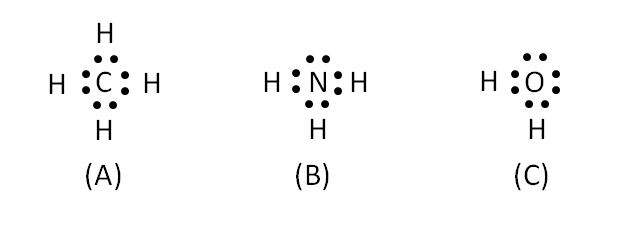Lewis dot diagram

Chemistry Level 2The above are Lewis dot diagrams of molecules (A), (B) and (C). Which of following is correct about these? (The atomic weights are $\ce H=1, \ce C=12, \ce N=14$)

a) The boiling point of (A) is lower than that of (B).

b) The bonding angle of (A) is larger than that of (C).

c) The solubility of (A) into liquid (C) is larger than that of (B).

×